The surface normal gradient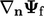is a part of the Laplacian discretisation Eq. (3.2 ), illustrated in the ﬁgure below.The discretisation of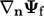is built upon a ﬁnite diﬀerence of cell values on each side of the face according to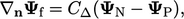(3.5)
where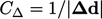. When this orthogonal scheme is applied to Eq. (3.2 ) to discretise a Laplacian, it forms coeﬃcients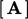of a matrix equation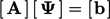since it references cell values of the ﬁeld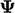. For cell, the coeﬃcient for each neighbour cell (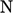) is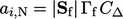and the diagonal coeﬃcient is the negative of the sum of neighbour coeﬃcients: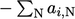.

Discretisation of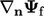by Eq. (3.5) is most accurate when the face is orthogonal to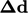, i.e. the angle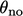between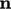andis zero. However, if the face is non-orthogonal, the error associated with Eq. (3.5 ) increases with.

### Non-orthogonal correction

A more accurate discretisation of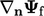at a non-orthogonal face is formed of the vector sum of the orthogonal scheme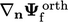and an explicit correction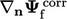. The latter is calculated from the full gradient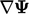in adjacent cells (described in Sec. 3.15 ), interpolated to the face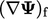.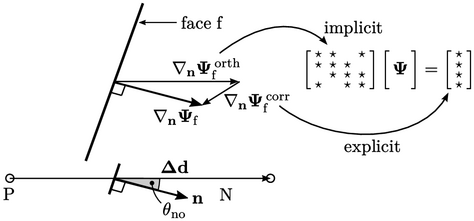The correction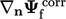is explicit, i.e. calculated using known values of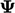, so may need updating within an iterative sequence to maintain accuracy, as discussed in Sec. 5.20 . To ensure that the iterative sequence converges, the implicit contribution is elevated by replacing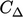in the orthogonal scheme with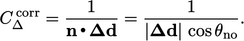(3.6)
The corrected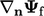scheme combines the implicit and explicit parts by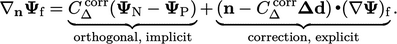(3.7)
The corrected scheme is generally stable for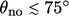. For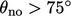, stability can be maintained at the expense of accuracy by limiting the magnitude of the correction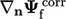below some fraction of the magnitude of the orthogonal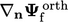part.
Notes on CFD: General Principles - 3.8 Surface normal gradient